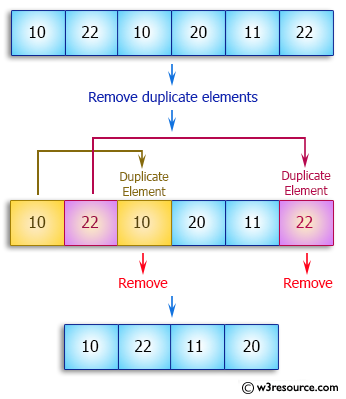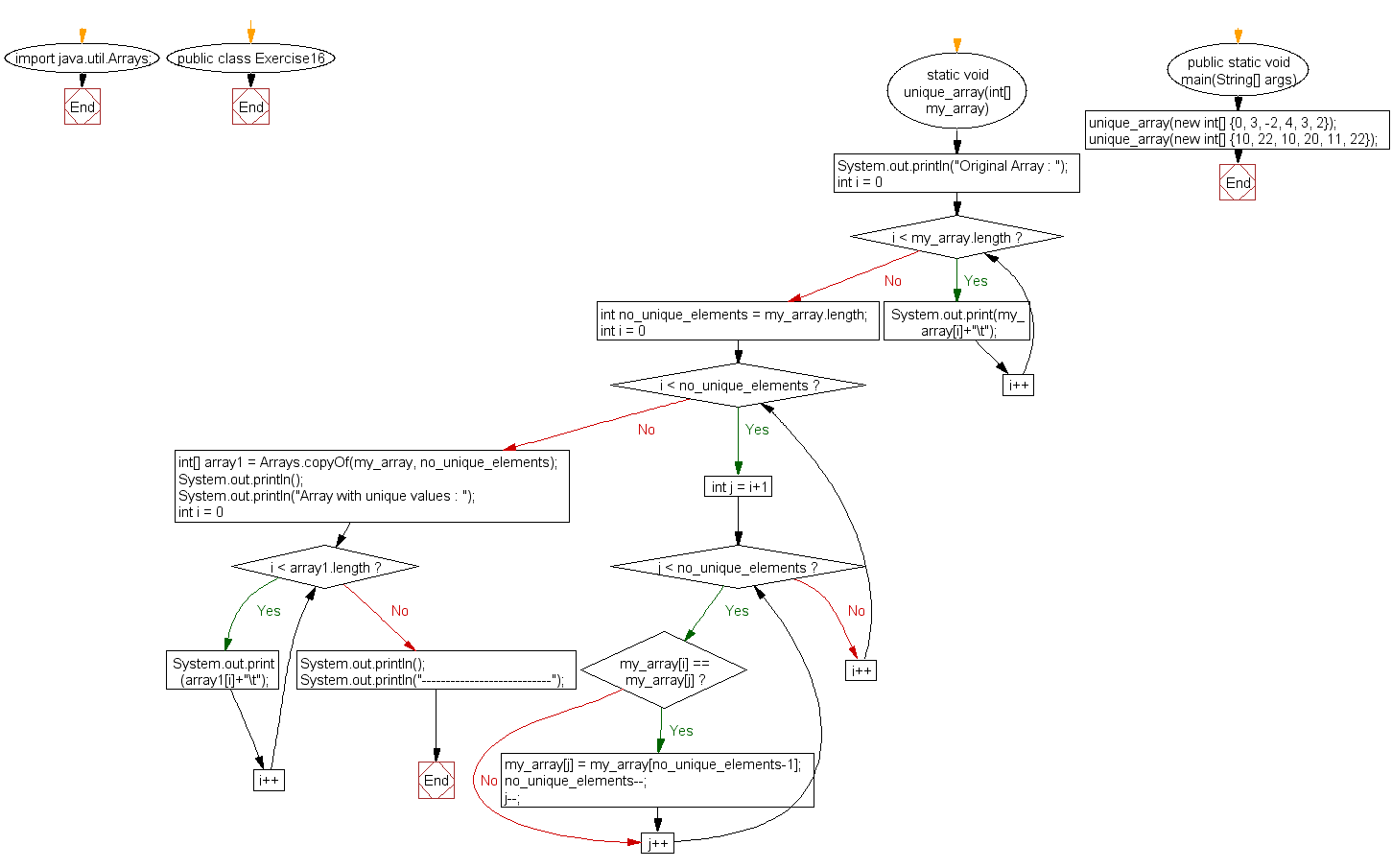﻿ Java exercises: Remove duplicate elements from an array - w3resource# Java Array Exercises: Remove duplicate elements from an array

## Java Array: Exercise-16 with Solution

Write a Java program to remove duplicate elements from an array.

Pictorial Presentation:Sample Solution:

Java Code:

``````import java.util.Arrays;

public class Exercise16 {
static void unique_array(int[] my_array)
{
System.out.println("Original Array : ");

for (int i = 0; i < my_array.length; i++)
{
System.out.print(my_array[i]+"\t");
}

//Assuming all elements in input array are unique

int no_unique_elements = my_array.length;

//Comparing each element with all other elements

for (int i = 0; i < no_unique_elements; i++)
{
for (int j = i+1; j < no_unique_elements; j++)
{
//If any two elements are found equal

if(my_array[i] == my_array[j])
{
//Replace duplicate element with last unique element

my_array[j] = my_array[no_unique_elements-1];

no_unique_elements--;

j--;
}
}
}

//Copying only unique elements of my_array into array1

int[] array1 = Arrays.copyOf(my_array, no_unique_elements);

//Printing arrayWithoutDuplicates

System.out.println();

System.out.println("Array with unique values : ");

for (int i = 0; i < array1.length; i++)
{
System.out.print(array1[i]+"\t");
}

System.out.println();

System.out.println("---------------------------");
}

public static void main(String[] args)
{
unique_array(new int[] {0, 3, -2, 4, 3, 2});

unique_array(new int[] {10, 22, 10, 20, 11, 22});

}
}
```
```

Sample Output:

```Original Array :
0       3       -2      4       3       2
Array with unique values :
0       3       -2      4       2
---------------------------
Original Array :
10      22      10      20      11      22
Array with unique values :
10      22      11      20
---------------------------
```

Flowchart:Visualize Java code execution (Python Tutor):

Java Code Editor:

Improve this sample solution and post your code through Disqus

What is the difficulty level of this exercise?

Test your Programming skills with w3resource's quiz.

﻿

## Java: Tips of the Day

countOccurrences

Counts the occurrences of a value in an array.

Use Arrays.stream().filter().count() to count total number of values that equals the specified value.

```public static long countOccurrences(int[] numbers, int value) {
return Arrays.stream(numbers)
.filter(number -> number == value)
.count();
}
```

Ref: https://bit.ly/3kCAgLb